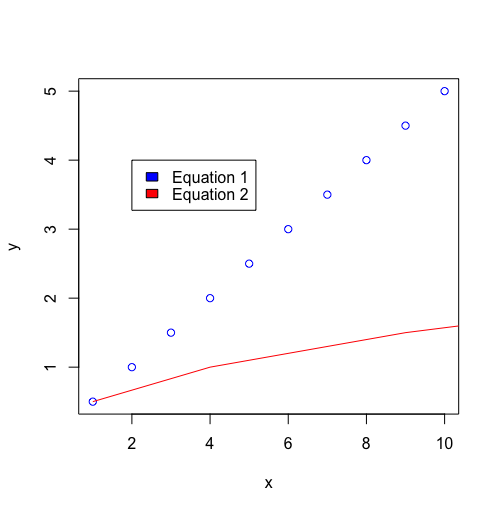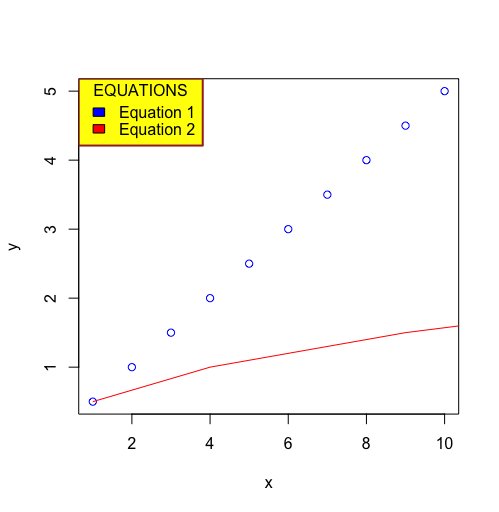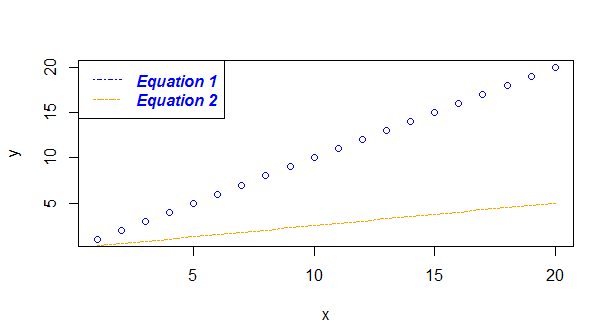# Add Legend to Plot in R

• Last Updated : 16 May, 2021

Legends are useful to add more information to the plots and enhance the user readability. It involves the creation of titles, indexes, placement of plot boxes in order to create a better understanding of the graphs plotted. The in-built R function legend() can be used to add legend to plot.

Syntax: legend(x, y, legend, fill, col, bg, lty, cex, title, text.font, bg)

Parameters:

• x and y: These are co-ordinates to be used to position the legend
• legend: Text of the legend
• fill: Colors to use for filling the boxes of legend text
• col: Colors of lines
• bg: It defines background color for the legend box.
• title: Legend title        (optional)
• text.font: An integer specifying the font style of the legend (optional)

Returns: Legend plot

After normally visualizing our plot, to add legend to it only appropriate arguments with required values are to be given to the legend() function.

Example:

## R

 `# declaring the data to plot ` `x<-1:10 ` `y=x^1/2 ` `z= x^2 ` ` `  `# plotting x and y coordinate  ` `# line  ` `plot``(x, y, col=``"blue"``) ` ` `  `# adding another line on the ` `# coordinates involving y and z ` `lines``(z, y ,col=``"red"``) ` ` `  `# Adding a legend to the graph ` `# defining the lines  ` `legend``(2, 4, legend=``c``(``"Equation 1"``, ``"Equation 2"``),  ` `       ``fill = ``c``(``"blue"``,``"red"``) ` `)`

OutputThe legend box in the graph can be customized to suit the requirements in order to convey more information and add aesthetics to the graph at the same time. Given below are properties of legends basing which they can be customized:

• title: The title of the legend box that can be declared to understand what the index of the indicates
• position :  Indicator of the placement of the legend box ; which can have the possible options : “bottomright”, “bottom”, “bottomleft”, “left”, “topleft”, “top”, “topright”, “right” and “center”
• bty (Default : o) : The type of box to enclose the legend . Different types of letters can be used, where the box shape is equivalent to the letter shape. For instance, “n” can be used for no box.
• bg: A background colour can be assigned to the legend box
• box.lwd : Indicator of the line width of the legend box
• box.lty : Indicator of the line type of the legend box
• box.col : Indicator of the line color of the legend box

When appropriate values are applied to these properties, and they are then passed to the legend() function the required customization is achieved.

Example:

## R

 `# declaring the data to plot ` `x<-1:10 ` `y=x^1/2 ` `z= x^2 ` ` `  `# plotting x and y coordinate line  ` `plot``(x, y, col=``"blue"``) ` ` `  `# adding another line on the  ` `# coordinates involving y and z ` `lines``(z, y ,col=``"red"``) ` ` `  `# Adding a legend to the graph ` `# defining the lines  ` `legend``(x = ``"topleft"``, box.col = ``"brown"``, ` `       ``bg =``"yellow"``, box.lwd = 2 , title=``"EQUATIONS"``,  ` `       ``legend=``c``(``"Equation 1"``, ``"Equation 2"``),  ` `       ``fill = ``c``(``"blue"``,``"red"``)) `

OutputThe text of the legend function can also be customized for better styling using the following properties:

• text.font: A numerical value which is an indicator of the font style of the legend text. It has the following values :(1 – normal 2- bold, 3 – italic, 4 – bold and italic)
• text.col : Which is used to indicate the color of the text used to write legend text
• border (Default : black) : Indicator of the border color of the boxes inside the legend box
• fill_color : colors used for filling boxes

Example:

## R

 `# declaring the data to plot ` `x<-20:1 ` `y=x ` `z= x*(1/4) ` ` `  `# plotting x and y coordinate line  ` `plot``(x, y, lty = 4,col=``"blue"``) ` ` `  `# adding another line on the  ` `# coordinates involving y and z ` `lines``(y, z ,lty = 6,col=``"orange"``) ` ` `  `# Adding a legend to the graph ` `# defining the lines  ` `legend``(x = ``"topleft"``, lty = ``c``(4,6), text.font = 4,  ` `       ``col= ``c``(``"blue"``,``"orange"``),text.col = ``"blue"``,  ` `       ``legend=``c``(``"Equation 1"``, ``"Equation 2"``)) `

OutputMy Personal Notes arrow_drop_up
Recommended Articles
Page :